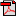# Lesson 2: Naming Fractions

## Getting Started

### Questions to Explore

• What is a fraction?
• What are the parts of a fraction?
• How do we write and speak in mathematical language?

### Facts and Definitions

• Numerator: the top number in a fraction, how many parts of the whole you have or need
• Denominator: the bottom number in a fraction, the total number of equal parts in a whole

### Skills

• Recognize parts of fractions: numerator and denominator
• Understand a fraction 1/b as the quantity formed by 1 part when a whole is partitioned into b equal parts
• Understand a fraction a/b as the quantity formed by a parts of size 1/b

### Materials

• A Fraction's Goal—Parts of a Whole by Brian P. Cleary
• colored pencils
• glue or glue stick
• scissors

### Introduction

Materials: scissors
Your child will cut out the cards on the "Matching Fractions" sheet and then create pairs that show the same fractions.
"Matching Fractions" Answer Key•Matching Fractions